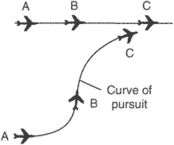# curve of pursuit

Also found in: Dictionary, Wikipedia.
The following article is from The Great Soviet Encyclopedia (1979). It might be outdated or ideologically biased.

## Curve of Pursuit

a plane curve that can be defined kinetically the following way. Suppose that a point P moves along the x-axis with a constant velocity a > 0 and that in the same plane a point M moves with a velocity v that is constant in absoluté value. If the velocity vector of M is always directed toward P,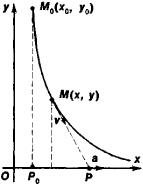Figure 1

the path M is called a curve of pursuit (Figure 1). If the coordinates of M are denoted by x and y, the differential equation of the curve of pursuit has the form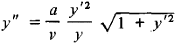where v= ǀvǀ.

Suppose that M0(x0, y0) and P0(x0, 0) are the positions of M and P, respectively, at the initial moment and that y0 > 0.

The equation of the curve of pursuit then has the form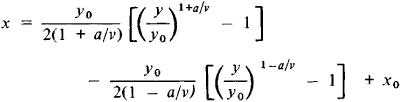when va, and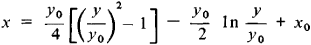when v = a. If v > a, y decreases from y0 to 0 as x increases from x0 to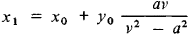that is, M catches up with P at the point x1 on the x-axis. In this case, the length of the curve of pursuit is equal to y0v2/(v2a2), and the time needed for M to catch up with P is T = y0v/(v2a2) (duration of pursuit). If v < a, M will not catch up with P.